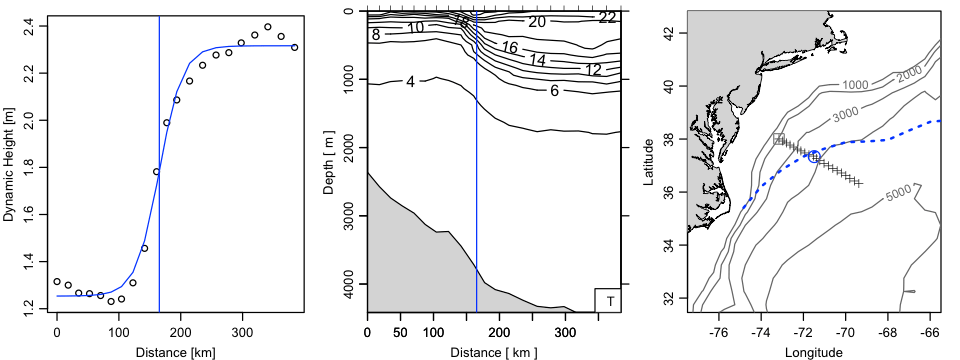Want to share your content on R-bloggers? click here if you have a blog, or here if you don't.

# Introduction

Definitions of Gulf Stream location sometimes centre on thermal signature, but it might make sense to work with dynamic height $\eta$ instead. This is illustrated here, using a $\tanh$ model for $\eta=\eta(x)$, with $x$ the distance along the transect. The idea is to select $x_ 0$, the halfway point in the $\tanh()$ function, where the slope is maximum and where therefore the inferred geostrophic velocity peaks.

# Methods and results

 1 library(oce) 
## Loading required package: methods

  1 2 3 4 5 6 7 8 9 10 11 12 13 14 15 16 17 18 19 20 21 22 23 24 25 26 27 28 29 30 31 32 33 34 35 36 37 38 39 40 41 data(section) ## Extract Gulf Stream (and reverse station order) GS <- subset(section, 109<=stationId & stationId<=129) GS <- sectionSort(GS, by="longitude") GS <- sectionGrid(GS) ## Compute and plot normalized dynamic height dh <- swDynamicHeight(GS) h <- dh$height x <- dh$distance par(mfrow=c(1, 3), mar=c(3, 3, 1, 1), mgp=c(2, 0.7, 0)) plot(x, h, xlab="Distance [km]", ylab="Dynamic Height [m]") ## Fit to tanh, with x0 line m <- nls(h~a+b*(1+tanh((x-x0)/L)), start=list(a=0,b=1,x0=100,L=100)) hp <- predict(m) lines(x, hp, col='blue') x0 <- coef(m)[["x0"]] abline(v=x0, col='blue') # Temperature section, again with x0 line plot(GS, which="temperature") abline(v=x0, col='blue') ## Show lon and lat of x0, on a map lon <- GS[["longitude", "byStation"]] lat <- GS[["latitude", "byStation"]] distance <- geodDist(lon, lat, alongPath=TRUE) lat0 <- approxfun(lat~distance)(x0) lon0 <- approxfun(lon~distance)(x0) plot(GS, which="map", map.xlim=lon0+c(-6,6), map.ylim=lat0+c(-6, 6)) points(lon0, lat0, pch=1, cex=2, col='blue') data(topoWorld) ## Show isobaths depth <- -topoWorld[["z"]] contour(topoWorld[["longitude"]]-360, topoWorld[["latitude"]], depth, level=1000*1:5, add=TRUE, col=gray(0.4)) ## Show Drinkwater September climatological North Wall of Gulf Stream. data("gs", package="ocedata") lines(gs$longitude, gs$latitude[,9], col='blue', lwd=2, lty='dotted')# Exercises

From the map, work out a scale factor for correcting geostrophic velocity from cross-section to along-stream, assuming the Drinkwater (1994) climatology to be relevant.

# Resources

• Source code: 2014-06-22-gulf-stream-center.R

• K. F. Drinkwater, R. A Myers, R. G. Pettipas and T. L. Wright, 1994.
Climatic data for the northwest Atlantic: the position of the shelf/slope
front and the northern boundary of the Gulf Stream between 50W and 75W,
1973-1992. Canadian Data Report of Fisheries and Ocean Sciences 125.
Department of Fisheries and Oceans, Canada.